Home
 Addition and Subtraction Materials: Deck of bars for each group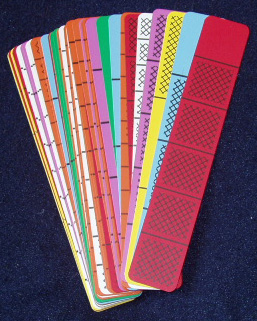Addition (combining the shaded parts of two bars) and subtraction (comparing the difference in shaded amounts of two bars) have been modeled in some of the earlier activities in this workshop. These activities help to show that the basic ideas of addition and subtraction are the same for fractions as for whole numbers, that is, the concepts do not change when we go from whole numbers to fractions. Combining to get total Comparing to find difference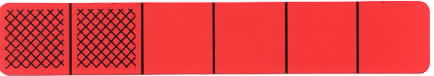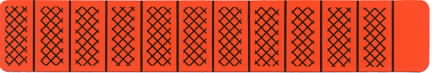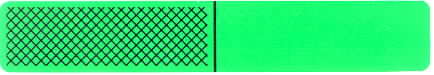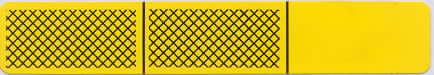2/6 + 1/2 = 5/6 11/12 - 2/3 = 3/12 Group Activities: a. Select two nonzero bars of different color and write an equation for their sum. b. Select two nonzero bars of different color and write an equation for their difference. . One method of computing the sum or difference of two fractions is to use visual models. Another method is to first find a common denominator for the fractions. It is then only necessary to compute the sum or difference of the numerators (whole numbers). Often we do not need an exact sum or difference and this is where the benchmarks of 1/2 and 1 become important for making estimations. Research shows that students often memorize fraction rules and addition and subtraction do not make sense. Put up the following transparency and discuss the NAEP highlights regarding students’ lack of conceptual knowledge of fractions.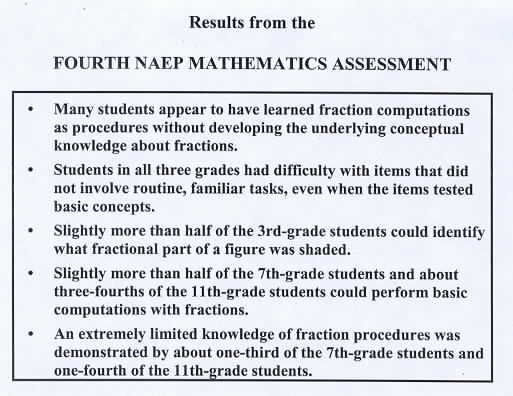NAEP Second Assessment Results Place the following NAEP results on the overhead and discuss. In particular, discuss the low performance by students in computing 1/2 + 1/3. Note that this question has been on most NAEP tests with fairly consistent results and about half of the students who answer this question incorrectly write the sum as 2/5.The next NAEP results require an estimation to 12/13 + 7/8. Ask for predictions as to why the incorrect answers of 19 and 21 were so frequently chosen.Discuss the need for visual models to help students see that 7/8 and 12/13 are both close to a whole. The variety of answers for the above question are very disturbing and indicate that when many students see fractions they only see symbols that do not make sense. For further activities involving addition and subtraction of fractions see Shading Blank Fraction Bars, Activity Sheet #4. This class is working in small groups. The students in the foreground are playing a version of Concentration; the total shaded amount of the two bars turned over must equal one whole bar.